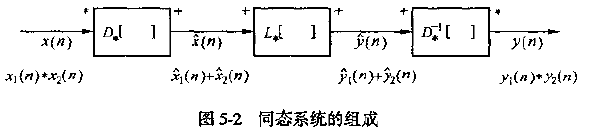22 篇文章 5 订阅

# 倒谱分析与同态滤波

## 同态信号处理的基本原理x(n)=x1(n)x2(n) x ( n ) = x 1 ( n ) ∗ x 2 ( n )

Z[x(n)]=X(z)=X1(z)X2(n) Z [ x ( n ) ] = X ( z ) = X 1 ( z ) X 2 ( n )

lnX(z)=lnX1(z)+lnX2(z)=X̂ 1(z)+X̂ 2(z)=X̂ (z) l n X ( z ) = l n X 1 ( z ) + l n X 2 ( z ) = X ^ 1 ( z ) + X ^ 2 ( z ) = X ^ ( z )

Z1[X̂ (z)]=Z1[X̂ 1(z)+X̂ 2(z)]=x̂ 1(n)+x̂ 2(n)=x̂ (n) Z − 1 [ X ^ ( z ) ] = Z − 1 [ X ^ 1 ( z ) + X ^ 2 ( z ) ] = x ^ 1 ( n ) + x ^ 2 ( n ) = x ^ ( n )

c(n)=F1[ln|X(ejw)|] c ( n ) = F − 1 [ l n | X ( e j w ) | ]

## 声门激励信号的复倒谱性质

x(n)=r=0Mαrδ(nrNp) x ( n ) = ∑ r = 0 M α r δ ( n − r N p )

x̂ (n)=lnα0δ(n)k=1[1kr=1Mαkrδ(nkNp)] x ^ ( n ) = l n α 0 δ ( n ) − ∑ k = 1 ∞ [ 1 k ∑ r = 1 M α r k δ ( n − k N p ) ]

• 有限长度周期冲激序列，其复倒谱也是周期冲激序列，且周期不变。利用该特性，可用高复倒谱窗从语音信号的复倒谱中提取浊音激励信号的特性，从而可用复倒谱提取基音
• 其振幅随k增大而衰减。

### 声道冲激响应序列的复倒谱性质

• x̂ (n) x ^ ( n ) $\hat{x}(n)$为双边序列，存在于 <n< − ∞ < n < ∞ $-\infty \lt n \lt \infty$范围内。
• x̂ (n) x ^ ( n ) $\hat{x}(n)$为衰减序列，随着 |n| | n | $|n|$的增大而减小。且其衰减速度比 1|n| 1 | n | $\frac{1}{|n|}$快，因此 x̂ (n) x ^ ( n ) $\hat{x}(n)$比x(n)更集中在原点附近。因而可以用低复倒谱窗在复倒谱域中提取声道冲击响应

# Ref

12-18
11-2087406-302635
11-06691
10-292255
03-266692
08-30147
04-011406
12-04296
07-093044
05-27901
01-14864
12-174999
03-2131
10-172万+
11-06637
02-251万+
10-13982点击重新获取扫码支付余额充值